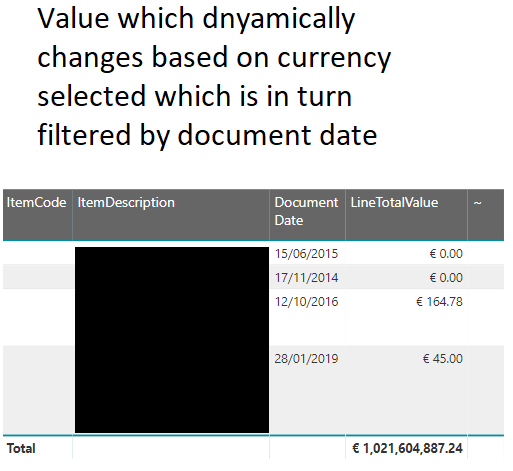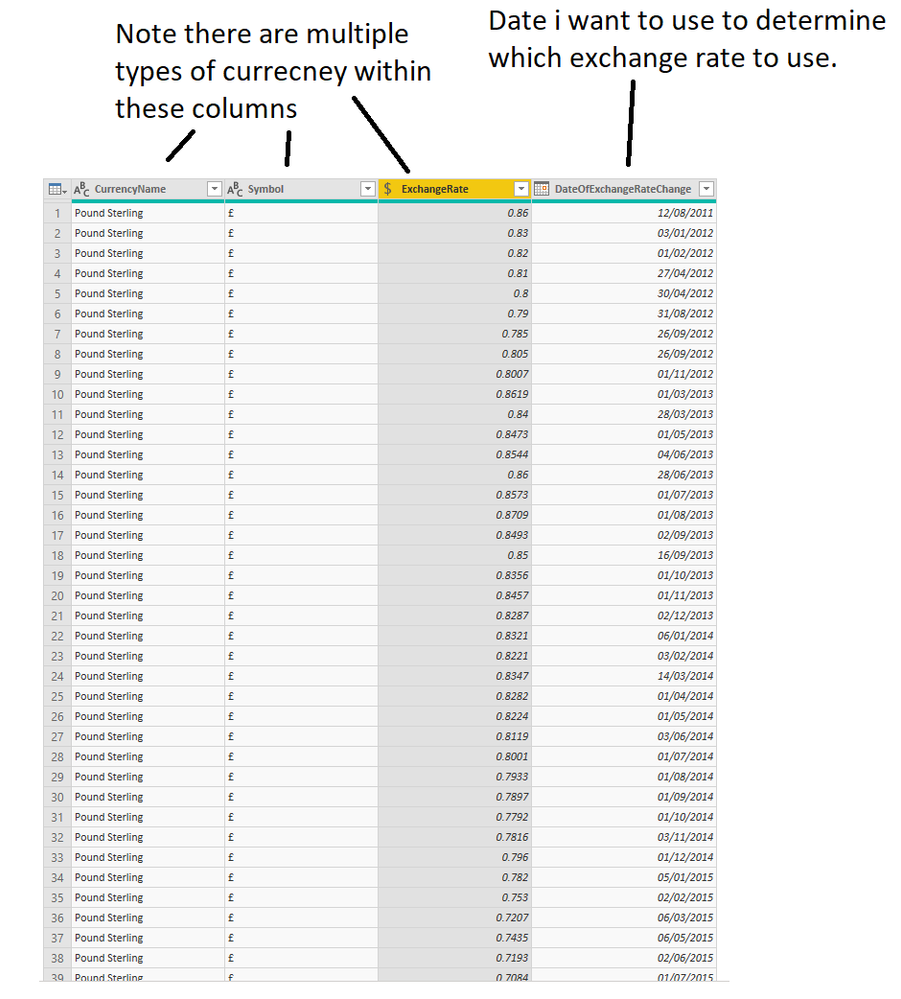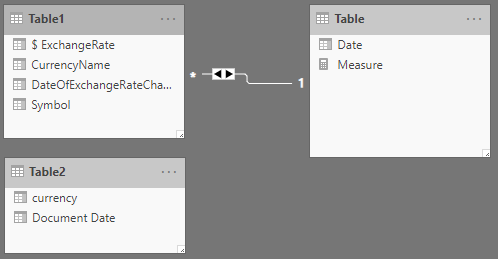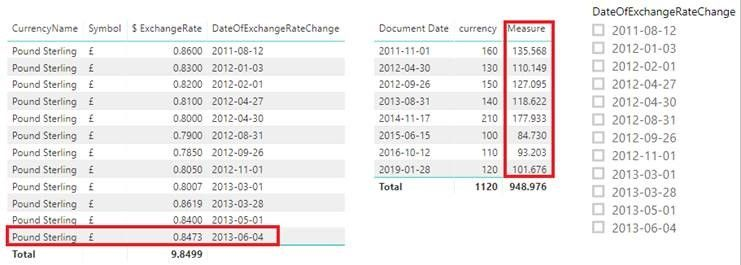cancel
Showing results for
Did you mean:Frequent Visitor

## Slicer which can change currency using an exchange rate which varies based on date

I have a table which contains multiple exchange rates these vary in terms of currency and date updated. Ultimately I want to create a measure which will allow me to convert values dynamically based on the currency I select in a filter. The exchange rates used to convert my values will in turn by based on the actual document date.

If a value in document date is more recent then a corresponding value within “DateOfExchangeRateChange” it should go to the next most recent date and if document date is more recent then the most recent value in “DateOfExchangeRateChange” it will instead use that last value.1 ACCEPTED SOLUTIONCommunity Support

Hi @DSArkphire ,

I created a sample that can be calculated at the current exchange rate with the selected date, or if it is not selected, based on the exchange rate of the latest date.

1. Create a calculated table.

`Table = CALENDARAUTO()`1. Create a measure

```Measure =
var a = SELECTEDVALUE('Table'[Date])
var b = CALCULATE(SUM(Table1[\$ExchangeRate])*SUM(Table2[currency]),
FILTER(Table1,Table1[DateOfExchangeRateChange] = a))
return
IF(ISFILTERED('Table'[Date]), b,
CALCULATE(SUM(Table1[\$ExchangeRate])*SUM(Table2[currency]),
FILTER('Table','Table'[Date] = MAX(Table1[DateOfExchangeRateChange]))))```Best Regards,

Xue Ding

If this post helps, then please consider Accept it as the solution to help the other members find it more quickly.

Best Regards,
Xue Ding
If this post helps, then please consider Accept it as the solution to help the other members find it more quickly.
2 REPLIES 2Community Support

Hi @DSArkphire ,

I created a sample that can be calculated at the current exchange rate with the selected date, or if it is not selected, based on the exchange rate of the latest date.

1. Create a calculated table.

`Table = CALENDARAUTO()`1. Create a measure

```Measure =
var a = SELECTEDVALUE('Table'[Date])
var b = CALCULATE(SUM(Table1[\$ExchangeRate])*SUM(Table2[currency]),
FILTER(Table1,Table1[DateOfExchangeRateChange] = a))
return
IF(ISFILTERED('Table'[Date]), b,
CALCULATE(SUM(Table1[\$ExchangeRate])*SUM(Table2[currency]),
FILTER('Table','Table'[Date] = MAX(Table1[DateOfExchangeRateChange]))))```Best Regards,

Xue Ding

If this post helps, then please consider Accept it as the solution to help the other members find it more quickly.

Best Regards,
Xue Ding
If this post helps, then please consider Accept it as the solution to help the other members find it more quickly.Anonymous
Not applicable

I need to do my requirement similar to this requirement but not able to get values by using given formula,

Can you pls see my details and do the needful pls , It would be great help if you can suggest on below

I need to show the data always 6 years of data from 2015  - 2021 till currency year dynamically

User will select the year slcier selection and based on the year selection actualcost will be multplied wiht the selected year currency rate  dynamically for all the year

Ex : if user select 2015 year in selection, 2015 rate will be applicable for all the 6 years ( cost should be multiplied with 2015 currency rate and if select 2017 then 2017 rate should be multiply with cost for all the 6 years

Matrix to be shown like below ( years will get changes dynamically in matrix and will be shown only 6 years back from currency year)

Basiness   location  2015   2016  2017  2018  2019  2020 2021

I have one data set with below columns :

Locationid

year

actualcost

currency id

exchange rate (with year wise)

no date in my dataset , only yearly and location base currency exchange rate are there in data set